# 第七节、双目视觉之空间坐标计算

$$M=\begin{bmatrix} f_x & γ & u_0 \\ 0 & f_y & v_0 \\ 0 & 0 & 1\end{bmatrix}$$

### 一、自己动手实现(只进行图片矫正、不进行立体校正)

1、标定双目后，首先要根据其畸变系数来矫正原图，这里用来去除图片的畸变：

        cv::Mat mapx = cv::Mat(imageSize, CV_32FC1);
cv::Mat mapy = cv::Mat(imageSize, CV_32FC1);
cv::Mat R = cv::Mat::eye(3, 3, CV_32F);
std::cout << "显示矫正图像" << endl;
for (int i = 0; i != imageCount; i++)
{
std::cout << "Frame #" << i + 1 << "..." << endl;
//计算图片畸变矫正的映射矩阵mapx、mapy(不进行立体校正，立体校正需要利用双摄)
initUndistortRectifyMap(cameraMatrix, distCoeffs, R, cameraMatrix, imageSize, CV_32FC1, mapx, mapy);
//读取一张图片
Mat newimage = imageSource.clone();
//另一种不需要转换矩阵的方式
//undistort(imageSource,newimage,cameraMatrix,distCoeffs);
//进行校正
remap(imageSource, newimage, mapx, mapy, INTER_LINEAR);
imshow("原始图像", imageSource);
imshow("矫正后图像", newimage);
waitKey();
}

2、坐标计算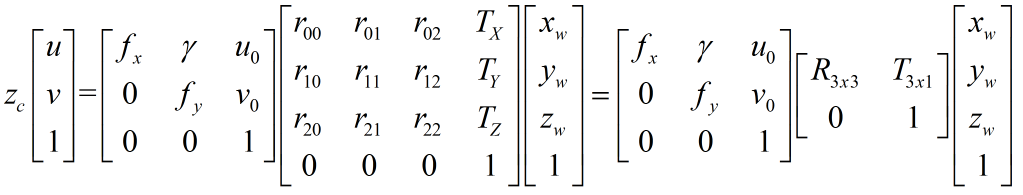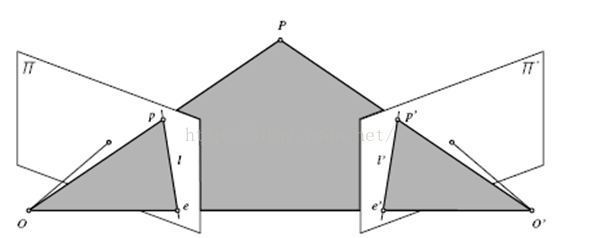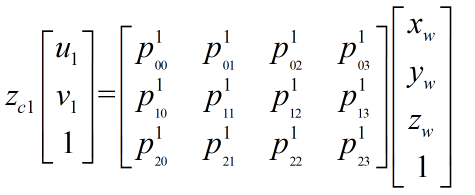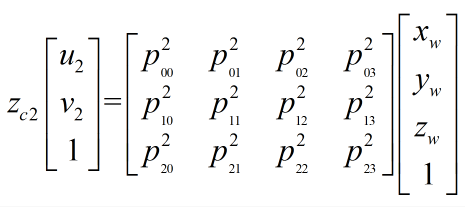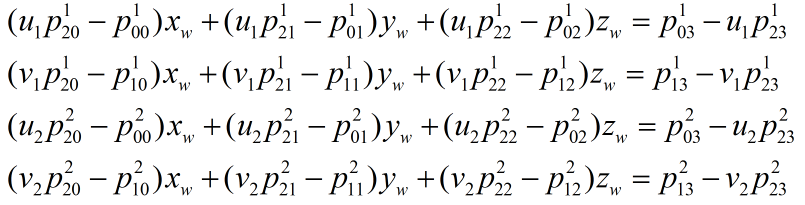//opencv2.4.9 vs2012
#include <opencv2\opencv.hpp>
#include <fstream>
#include <iostream>

using namespace std;
using namespace cv;

Point2f xyz2uv(Point3f worldPoint,float intrinsic,float translation,float rotation);
Point3f uv2xyz(Point2f uvLeft,Point2f uvRight);

//图片对数量
int PicNum = 14;

//左相机内参数矩阵
float leftIntrinsic = {4037.82450,             0,        947.65449,
0,    3969.79038,        455.48718,
0,             0,                1};
//左相机畸变系数
float leftDistortion = {0.18962, -4.05566, -0.00510, 0.02895, 0};
//左相机旋转矩阵
float leftRotation = {0.912333,        -0.211508,         0.350590,
0.023249,        -0.828105,        -0.560091,
0.408789,         0.519140,        -0.750590};
//左相机平移向量
float leftTranslation = {-127.199992, 28.190639, 1471.356768};

//右相机内参数矩阵
float rightIntrinsic = {3765.83307,             0,        339.31958,
0,    3808.08469,        660.05543,
0,             0,                1};
//右相机畸变系数
float rightDistortion = {-0.24195, 5.97763, -0.02057, -0.01429, 0};
//右相机旋转矩阵
float rightRotation = {-0.134947,         0.989568,        -0.050442,
0.752355,         0.069205,        -0.655113,
-0.644788,        -0.126356,        -0.753845};
//右相机平移向量
float rightTranslation = {50.877397, -99.796492, 1507.312197};

int main()
{
//已知空间坐标求像素坐标
Point3f point(700,220,530);
cout<<"左相机中坐标："<<endl;
cout<<xyz2uv(point,leftIntrinsic,leftTranslation,leftRotation)<<endl;
cout<<"右相机中坐标："<<endl;
cout<<xyz2uv(point,rightIntrinsic,rightTranslation,rightRotation)<<endl;

//已知左右相机像素坐标求空间坐标
Point2f l = xyz2uv(point,leftIntrinsic,leftTranslation,leftRotation);
Point2f r = xyz2uv(point,rightIntrinsic,rightTranslation,rightRotation);
Point3f worldPoint;
worldPoint = uv2xyz(l,r);
cout<<"空间坐标为:"<<endl<<uv2xyz(l,r)<<endl;

system("pause");

return 0;
}

//************************************
// Description: 根据左右相机中像素坐标求解空间坐标
// Method:    uv2xyz
// FullName:  uv2xyz
// Access:    public
// Parameter: Point2f uvLeft
// Parameter: Point2f uvRight
// Returns:   cv::Point3f
// Author:    小白
// Date:      2017/01/10
// History:
//************************************
Point3f uv2xyz(Point2f uvLeft,Point2f uvRight)
{
//     [u1]      [xw]                      [u2]      [xw]
//zc1 *|v1| = Pl*[yw]                  zc2*|v2| = P2*[yw]
//     [ 1]      [zw]                      [ 1]      [zw]
//               [1 ]                                [1 ]
Mat mLeftRotation = Mat(3,3,CV_32F,leftRotation);
Mat mLeftTranslation = Mat(3,1,CV_32F,leftTranslation);
Mat mLeftRT = Mat(3,4,CV_32F);//左相机RT矩阵
hconcat(mLeftRotation,mLeftTranslation,mLeftRT);
Mat mLeftIntrinsic = Mat(3,3,CV_32F,leftIntrinsic);
Mat mLeftP = mLeftIntrinsic * mLeftRT;
//cout<<"左相机P矩阵 = "<<endl<<mLeftP<<endl;

Mat mRightRotation = Mat(3,3,CV_32F,rightRotation);
Mat mRightTranslation = Mat(3,1,CV_32F,rightTranslation);
Mat mRightRT = Mat(3,4,CV_32F);//右相机RT矩阵
hconcat(mRightRotation,mRightTranslation,mRightRT);
Mat mRightIntrinsic = Mat(3,3,CV_32F,rightIntrinsic);
Mat mRightP = mRightIntrinsic * mRightRT;
//cout<<"右相机P矩阵 = "<<endl<<mRightP<<endl;

//最小二乘法A矩阵
Mat A = Mat(4,3,CV_32F);
A.at<float>(0,0) = uvLeft.x * mLeftP.at<float>(2,0) - mLeftP.at<float>(0,0);
A.at<float>(0,1) = uvLeft.x * mLeftP.at<float>(2,1) - mLeftP.at<float>(0,1);
A.at<float>(0,2) = uvLeft.x * mLeftP.at<float>(2,2) - mLeftP.at<float>(0,2);

A.at<float>(1,0) = uvLeft.y * mLeftP.at<float>(2,0) - mLeftP.at<float>(1,0);
A.at<float>(1,1) = uvLeft.y * mLeftP.at<float>(2,1) - mLeftP.at<float>(1,1);
A.at<float>(1,2) = uvLeft.y * mLeftP.at<float>(2,2) - mLeftP.at<float>(1,2);

A.at<float>(2,0) = uvRight.x * mRightP.at<float>(2,0) - mRightP.at<float>(0,0);
A.at<float>(2,1) = uvRight.x * mRightP.at<float>(2,1) - mRightP.at<float>(0,1);
A.at<float>(2,2) = uvRight.x * mRightP.at<float>(2,2) - mRightP.at<float>(0,2);

A.at<float>(3,0) = uvRight.y * mRightP.at<float>(2,0) - mRightP.at<float>(1,0);
A.at<float>(3,1) = uvRight.y * mRightP.at<float>(2,1) - mRightP.at<float>(1,1);
A.at<float>(3,2) = uvRight.y * mRightP.at<float>(2,2) - mRightP.at<float>(1,2);

//最小二乘法B矩阵
Mat B = Mat(4,1,CV_32F);
B.at<float>(0,0) = mLeftP.at<float>(0,3) - uvLeft.x * mLeftP.at<float>(2,3);
B.at<float>(1,0) = mLeftP.at<float>(1,3) - uvLeft.y * mLeftP.at<float>(2,3);
B.at<float>(2,0) = mRightP.at<float>(0,3) - uvRight.x * mRightP.at<float>(2,3);
B.at<float>(3,0) = mRightP.at<float>(1,3) - uvRight.y * mRightP.at<float>(2,3);

Mat XYZ = Mat(3,1,CV_32F);
//采用SVD最小二乘法求解XYZ
solve(A,B,XYZ,DECOMP_SVD);

//cout<<"空间坐标为 = "<<endl<<XYZ<<endl;

//世界坐标系中坐标
Point3f world;
world.x = XYZ.at<float>(0,0);
world.y = XYZ.at<float>(1,0);
world.z = XYZ.at<float>(2,0);

return world;
}

//************************************
// Description: 将世界坐标系中的点投影到左右相机像素坐标系中
// Method:    xyz2uv
// FullName:  xyz2uv
// Access:    public
// Parameter: Point3f worldPoint
// Parameter: float intrinsic
// Parameter: float translation
// Parameter: float rotation
// Returns:   cv::Point2f
// Author:    小白
// Date:      2017/01/10
// History:
//************************************
Point2f xyz2uv(Point3f worldPoint,float intrinsic,float translation,float rotation)
{
//    [fx γ u0]                            [xc]        [xw]        [u]       [xc]
//M = |0 fy v0|       TEMP = [R T]         |yc| = TEMP*[yw]     zc*[v] =   M*[yc]
//    [ 0 0 1 ]                            [zc]        [zw]               [zc]
//                                                     [1 ]
Point3f c;
c.x = rotation*worldPoint.x + rotation*worldPoint.y + rotation*worldPoint.z + translation*1;
c.y = rotation*worldPoint.x + rotation*worldPoint.y + rotation*worldPoint.z + translation*1;
c.z = rotation*worldPoint.x + rotation*worldPoint.y + rotation*worldPoint.z + translation*1;

Point2f uv;
uv.x = (intrinsic*c.x + intrinsic*c.y + intrinsic*c.z)/c.z;
uv.y = (intrinsic*c.x + intrinsic*c.y + intrinsic*c.z)/c.z;

return uv;
}


### 二、利用OpenCV库实现(进行图片矫正、立体校正)

#### 1、双目立体标定

$$P_l = R_lP_w+T_l$$

$$R_r=R_rP_w+T_r$$

$$P_r = R_rR_l^{-1}(P_l-T_l)+T_r=R_rR_l^{-1}P_l+T_r-R_rR_l^{-1}T_l$$

$$R=R_rR_l^{-1}=R_rR_l^T$$

$$T=T_r-R_rR_l^{-1}T_l$$

#### 2、立体校正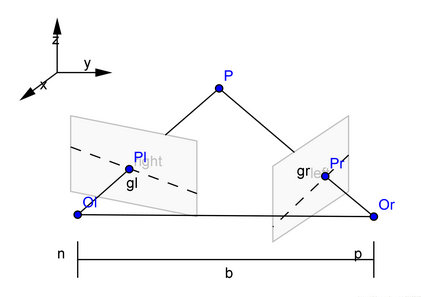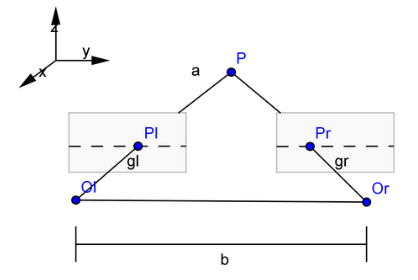#### 3、Bouguet校正原理

Bouguet的方法，是将旋转矩阵$R$和平移矩阵$T$分解成左右相机各旋转一半的旋转和平移矩阵$R_l,T_l,R_r,T_r$分解的原则是使得，左右图像重投影造成的畸变最小，左右视图的共同面积最大。

1、将右图像平面相对于左图像平面的旋转矩阵分解成两个矩阵$R_l$和$R_r$，叫做左右相机的合成旋转矩阵。

$$R_l=R^{\frac{1}{2}}$$

$$R_r=R^{-\frac{1}{2}}$$

2、将左右相机各旋转一半，使得左右相机的光轴平行。此时左右相机的成像面达到平行，但是基线与成像平面不平行。

3、构造变换矩矩阵$R_{rect}$使得基线与成像平面平行。构造的方法是通过右相机相对于左相机的偏移矩阵$T$完成的。

• 构造$e_1$。变换矩阵将左视图的极点变换到无穷远处，则使极线达到水平，可见，左右相机的投影中心之间的平移向量就是左极点方向：

$$e_1=\frac{T}{\| T\|}，T={\begin{bmatrix} T_x & T_y & T_z\end{bmatrix}}^T$$

• $e_2$方向与主光轴方向正交，沿图像方向，与$e_1$垂直，则知$e_2$方向可通过$e_1$与主光轴方向的叉积并归一化获得

$$e_2=\frac{\begin{bmatrix}-T_y & T_x & 0 \end{bmatrix}}{\sqrt{T_x^2+T_y^2}}$$

• 获取了$e_1$与$e_2$后，$e_3$与$e_1$和$e_2$正交，$e_1$自然就是他们两个的叉积：

$$e_3=e_1\times{e_2}$$

• 则可将左相机的极点转换到无穷远处的矩阵$R_{rect}$,如下：

$$R_{rect}=\begin{bmatrix} e_1^T \\ e_2^T \\ e_3^T \end{bmatrix}$$

• 通过合成旋转矩阵与变换矩阵相乘获得左右相机的整体旋转矩阵。左右相机坐标系乘以各自的整体旋转矩阵就可使得左右相机的主光轴平行，且像平面与基线平行。

$$R_l'=R_{rect}R_l，R_r'=R_{rect}R_r$$

• 通过上述的两个整体旋转矩阵，就能够得到理想的平行配置的双目立体系图像。校正后根据需要对图像进行裁剪，需重新选择一个图像中心，和图像边缘从而让左、右叠加部分最大。

#### 4、stereoCalibrate 函数

OpenCV提供了函数cvStereoCalibrate，用来进行双目标定，直接通过stereoCalibrate来实现双目定标，很容易产生比较大的图像畸变，边角处的变形较厉害。最好先通过calibrateCamera() 对每个摄像头单独进行定标，再利用stereoCalibrate进行双目定标。这样定标所得参数才比较准确，随后的校正也不会有明显的畸变。(注意：opencv提供的立体标定函数稳定性不太好，而且精度相对来言也不准，推荐使用matlab的立体标定)

/*
进行立体双目标定
由于左右摄像机分别都经过了单目标定
所以在此处选择flag = CALIB_USE_INTRINSIC_GUESS
*/
double rms = stereoCalibrate(objRealPoint,   //vector<vector<point3f>> 型的数据结构，存储标定角点在世界坐标系中的位置
imagePointL,                             //vector<vector<point2f>> 型的数据结构，存储标定角点在第一个摄像机下的投影后的亚像素坐标
imagePointR,                             //vector<vector<point2f>> 型的数据结构，存储标定角点在第二个摄像机下的投影后的亚像素坐标
cameraMatrixL,                           //输入/输出型的第一个摄像机的相机矩阵。如果CV_CALIB_USE_INTRINSIC_GUESS , CV_CALIB_FIX_ASPECT_RATIO ,CV_CALIB_FIX_INTRINSIC , or CV_CALIB_FIX_FOCAL_LENGTH其中的一个或多个标志被设置，该摄像机矩阵的一些或全部参数需要被初始化
distCoeffL,                              //第一个摄像机的输入/输出型畸变向量。根据矫正模型的不同，输出向量长度由标志决定
cameraMatrixR,                           //输入/输出型的第二个摄像机的相机矩阵。参数意义同第一个相机矩阵相似
distCoeffR,                              //第一个摄像机的输入/输出型畸变向量。根据矫正模型的不同，输出向量长度由标志决定
Size(imageWidth, imageHeight),           //图像的大小
R,                                       //输出型，第一和第二个摄像机之间的旋转矩阵
T,                                       //输出型，第一和第二个摄像机之间的平移矩阵
E,                                       //输出本征矩阵
F,                                       //输出基础矩阵
CALIB_USE_INTRINSIC_GUESS,
TermCriteria(TermCriteria::COUNT + TermCriteria::EPS, 100, 1e-5));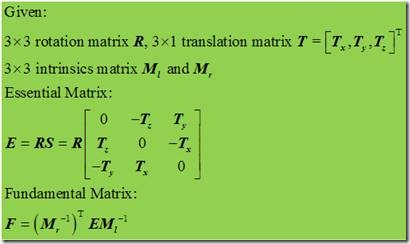stereoCalibrate 是怎样计算 Essential Matrix 和 Fundamental Matrix 的？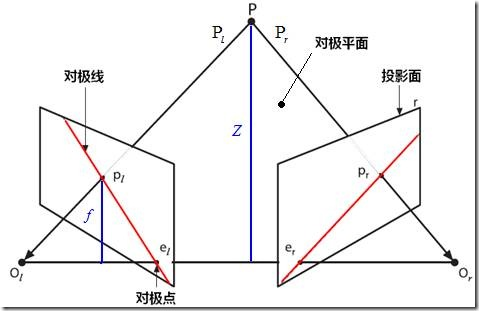（1）Essential Matrix

$$P_{cr}=R(P_{cl}-T)$$

$$P_{cl}-T=R^{-1}P_{cr}$$

$$(P_{cl}-T)^T=(R^{-1}P_{cr})^T=(R^TP_{cr})^T$$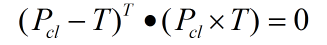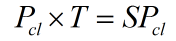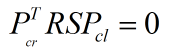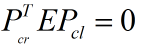（2）Fundamental Matrix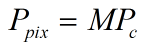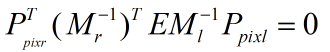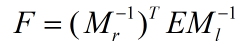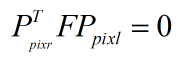#### 5、 stereoRectify函数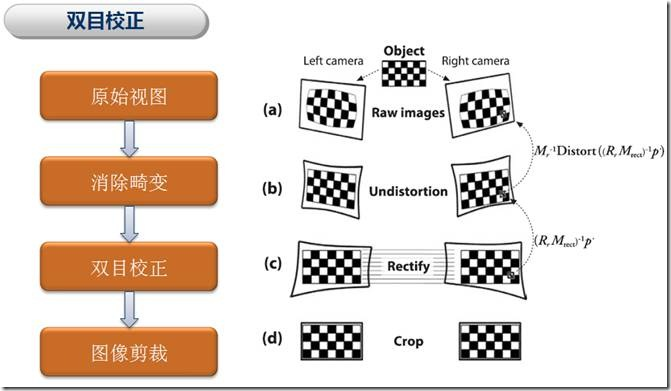如上图所示：双目校正是根据摄像头定标后获得的单目内参数据（焦距、成像原点、畸变系数）和双目相对位置关系（旋转矩阵和平移向量），分别对左右视图进行消除畸变和行对准，使得左右视图的成像原点坐标一致（CV_CALIB_ZERO_DISPARITY 标志位设置时发生作用）、两摄像头光轴平行、左右成像平面共面、对极线行对齐。在OpenCV2.1版之前，cvStereoRectify 的主要工作就是完成上述操作，校正后的显示效果如上图(c) 所示。可以看到校正后左右视图的边角区域是不规则的，而且对后续的双目匹配求取视差会产生影响，因为这些边角区域也参与到匹配操作中，其对应的视差值是无用的、而且一般数值比较大，在三维重建和机器人避障导航等应用中会产生不利影响。

CV_EXPORTS_W void stereoRectify( InputArray cameraMatrix1, InputArray distCoeffs1,
InputArray cameraMatrix2, InputArray distCoeffs2,
Size imageSize, InputArray R, InputArray T,
OutputArray R1, OutputArray R2,
OutputArray P1, OutputArray P2,
OutputArray Q, int flags = CALIB_ZERO_DISPARITY,
double alpha = -1, Size newImageSize = Size(),
CV_OUT Rect* validPixROI1 = 0, CV_OUT Rect* validPixROI2 = 0 );

（1）newImgSize：校正后remap图像的分辨率。如果输入为(0,0)，则是与原图像大小一致。对于图像畸变系数比较大的，可以把newImgSize 设得大一些，以保留图像细节(你可以把畸变矫正的过程想象成和图片旋转类似，在图片旋转中可能会出现边角超出当前图像，因此为了保留所有的图片信息，我们可以利用公式计算出旋转之后的面积，然后再进行旋转变换，这里畸变矫正也可能存在同样的问题，但是这个大小并不好计算，因此我们可以人为的设置大一些)。

（2）alpha：图像剪裁系数，取值范围是-1、0~1。当取值为 0 时，OpenCV会对校正后的图像进行缩放和平移，使得remap图像只显示有效像素（即去除不规则的边角区域），如下图，适用于机器人避障导航等应用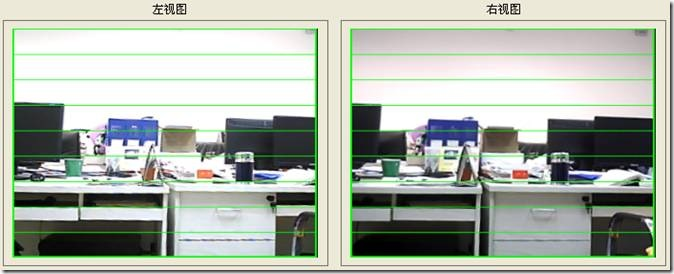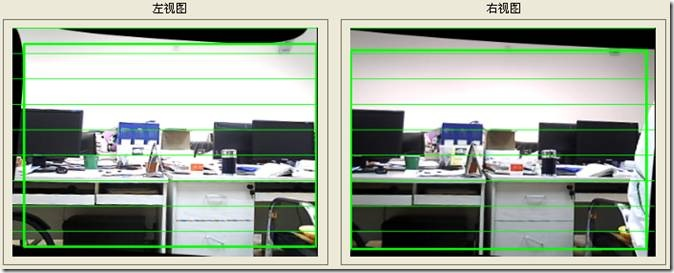（3）roi1, roi2：用于标记remap图像中包含有效像素的矩形区域。

$P1$,$P2$分别为左右相机的投影矩阵，其作用是将世界坐标系的点转换到像素坐标系下(左相机光心为世界坐标系原点):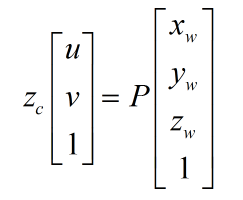$Q$矩阵为重投影矩阵，即矩阵$Q$可以把像素坐标系下的点转换到世界坐标系下: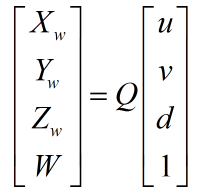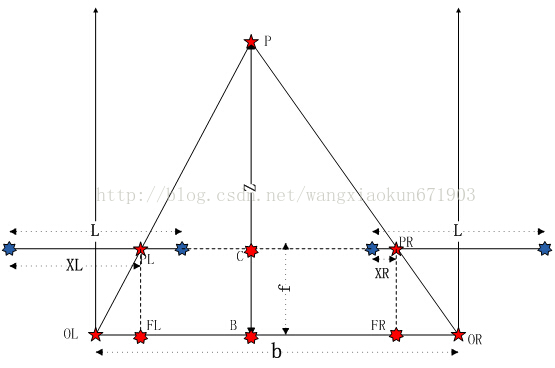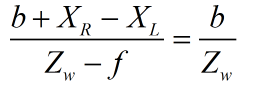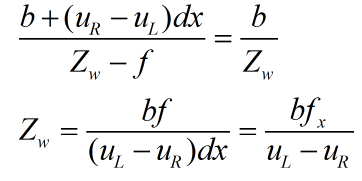$u_R$,$u_L$分别表示$P_L$、$P_R$距离各自像平面左边缘的像素距离。$f_x$是我们通过相机标定的内参值。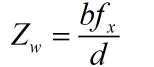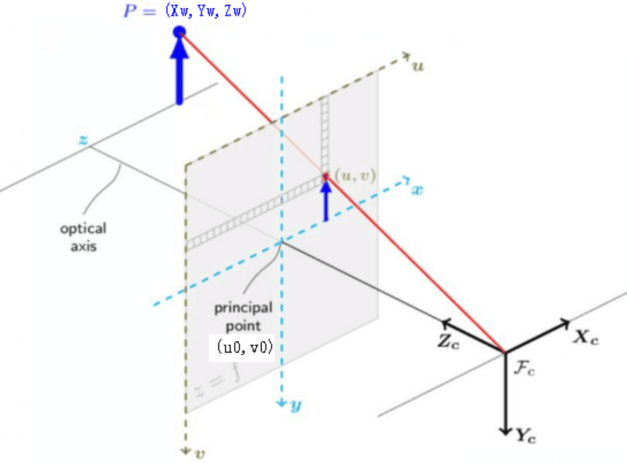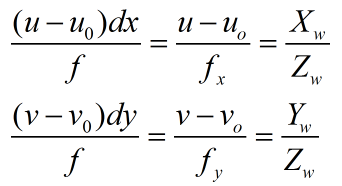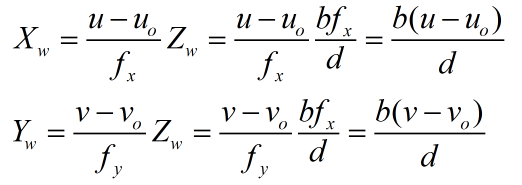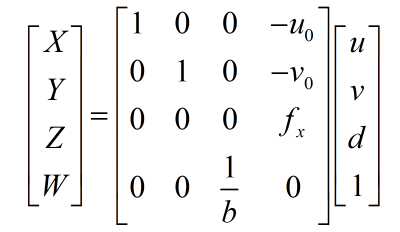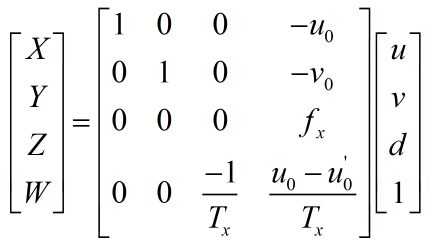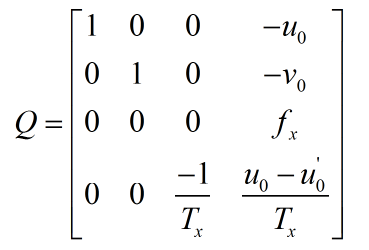#### 6、initUndistortRectifyMap函数

    /*
根据stereoRectify 计算出来的R 和 P 来计算畸变矫正和立体校正的映射变换矩阵 mapx,mapy
mapx,mapy这两个映射表接下来可以给remap()函数调用，来校正图像，使得两幅图像共面并且行对准
*/
initUndistortRectifyMap(cameraMatrixL, distCoeffL, Rl, Pl, imageSize, CV_32FC1, mapLx, mapLy);
initUndistortRectifyMap(cameraMatrixR, distCoeffR, Rr, Pr, imageSize, CV_32FC1, mapRx, mapRy);

### 三 立体匹配算法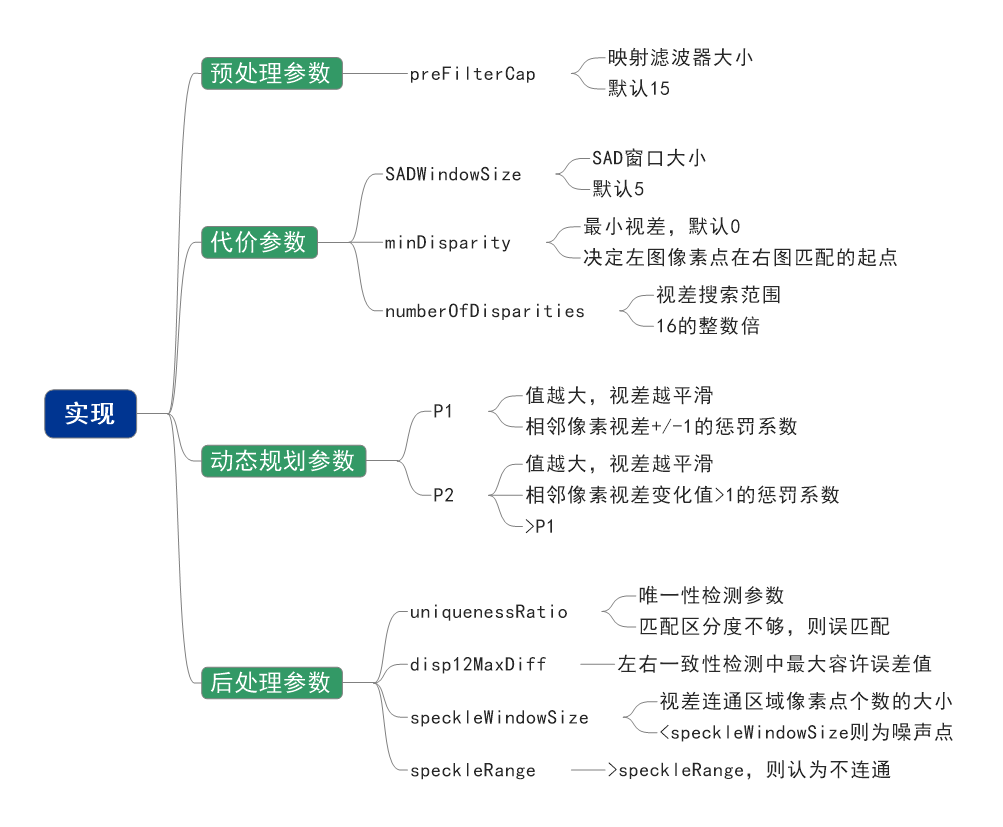### 四 程序讲解

• calibration.cpp：相机单目标定；
• stereo_calib.cpp：相机双目标定；
• stereo_match.cpp：相机立体匹配；

#### 1、标准数据集测试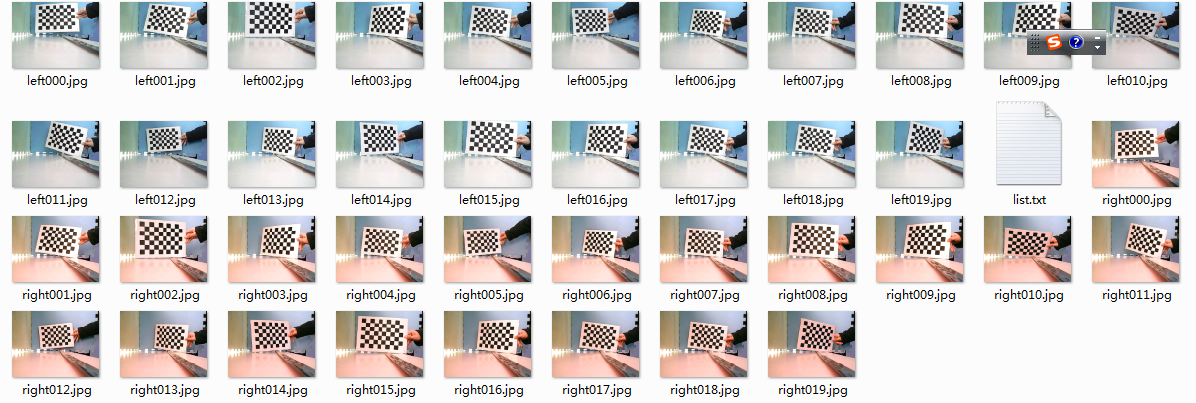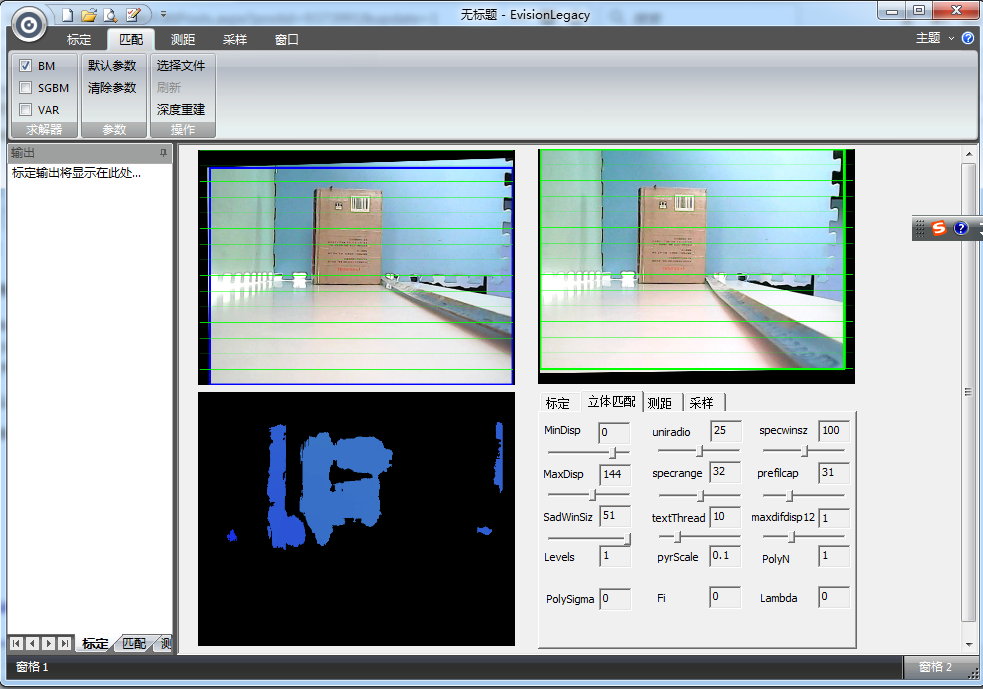1、我们尝试修改uniquenessRatio参数分别为5，15，25，结果如下：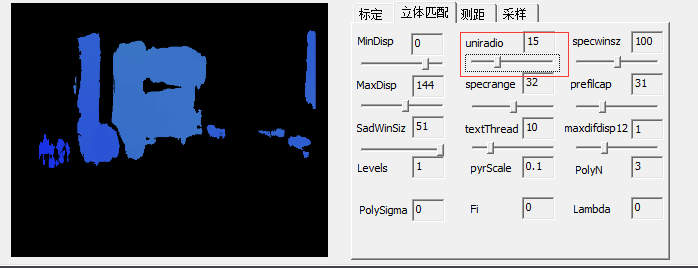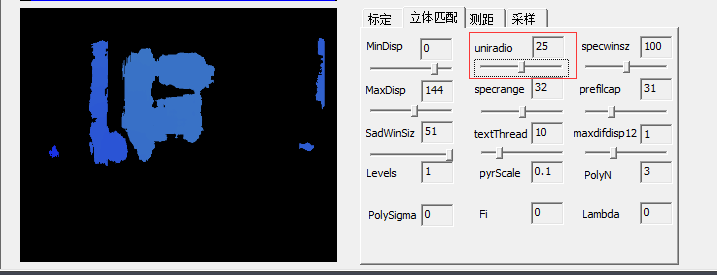uniquenessRatio主要可以防止误匹配，其主要作用从上面三幅图的视差效果比对就可以看出。在立体匹配中，我们宁愿区域无法匹配，也不要误匹配。如果有误匹配的话，碰到障碍检测这种应用，就会很麻烦。

2、我们尝试修改windowSize参数分别为5,25,51,结果如下：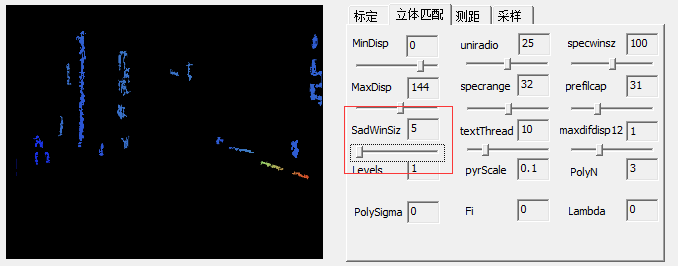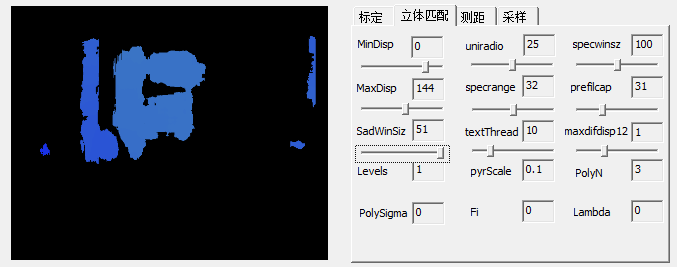3.我们尝试修改numberOfDisparities参数为16、32、64、128、256，结构如下：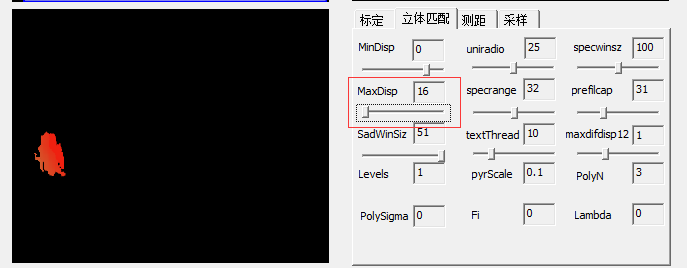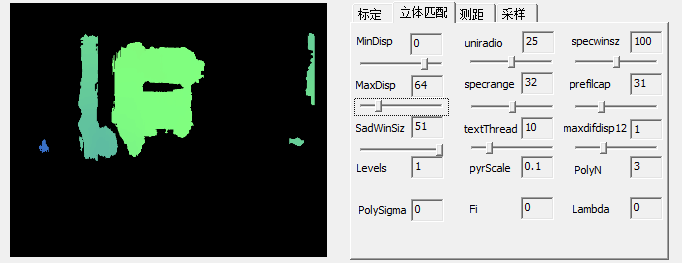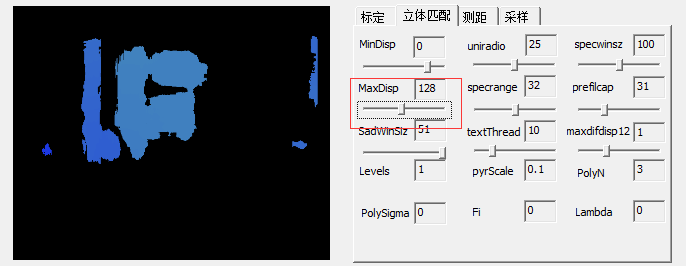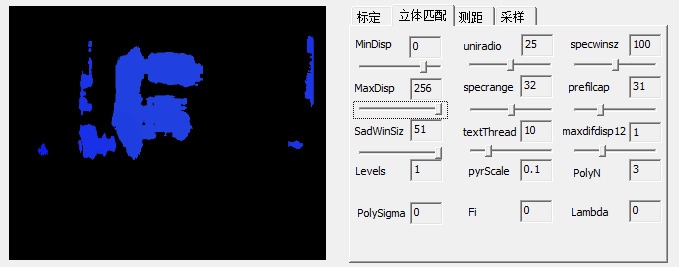numberOfDisparities表示视差窗口，即最大视差值与最小视差值之差, 窗口大小必须是 16 的整数倍，我们上面设置的默认最小视差为0，那么最大视差就为0+numberOfDisparities。从上面可以看到该值的影响很大。

下面我们看一下，测距效果，我们点击深度重建，这里我们利用二值化把前景和背景分离，然后利用 findContours()函数查找视差图的轮廓，并用最小矩形圈出来，效果如下：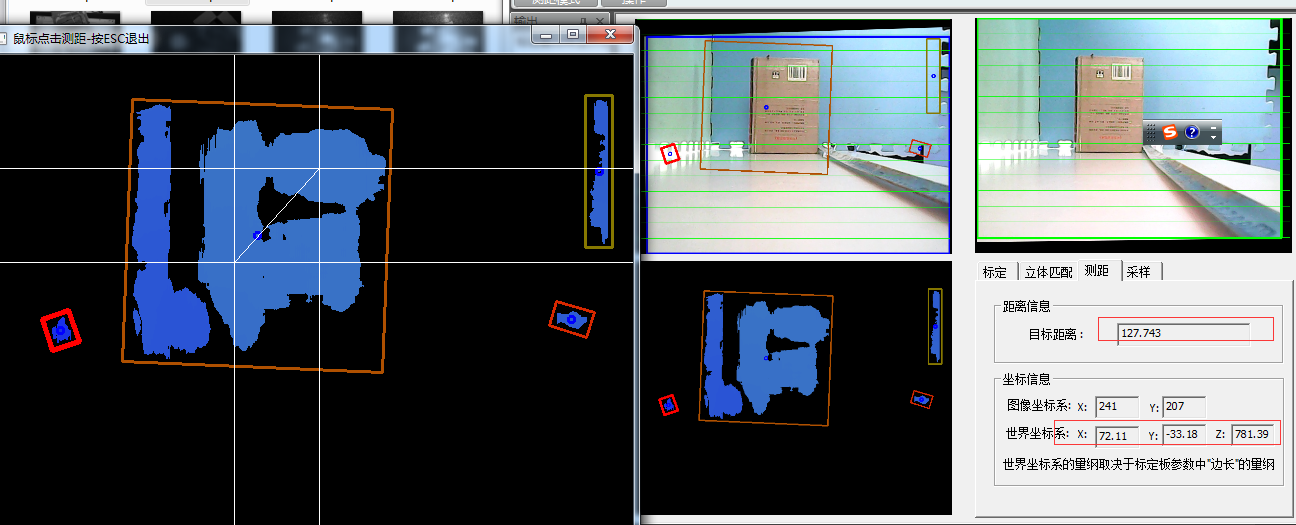#### 2、basler相机数据集

我利用实验室的两个balser相机拍摄了几十张用来标定的图片如下：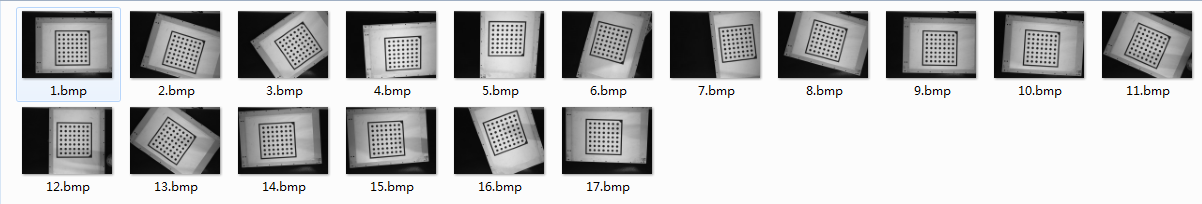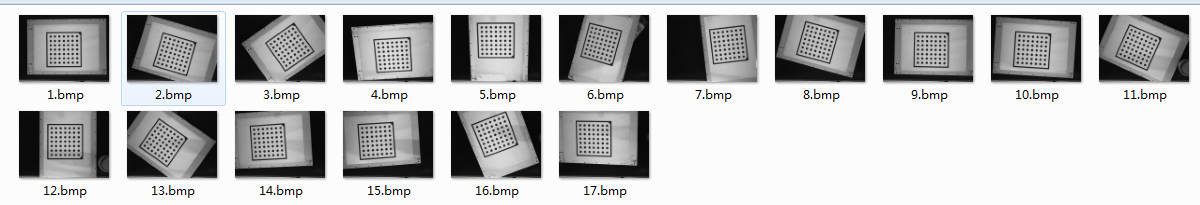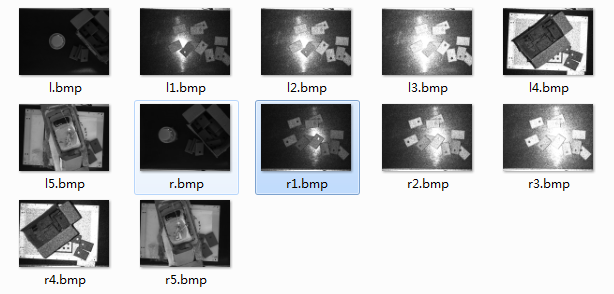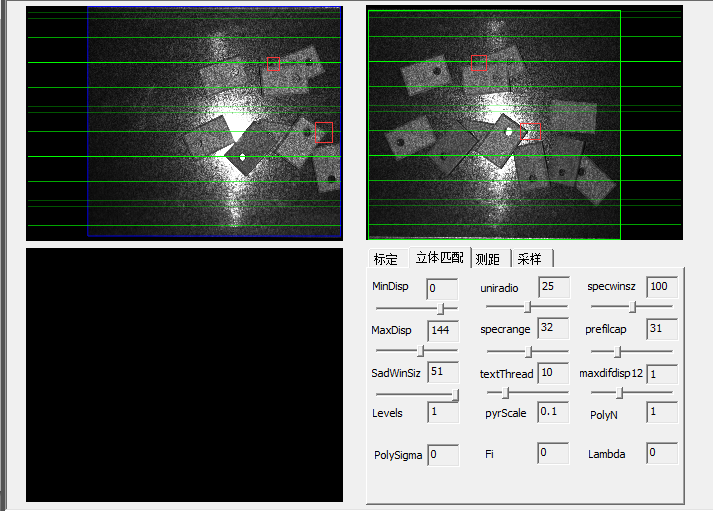（1） 光学失真和噪声（亮度、色调、饱和度等失衡）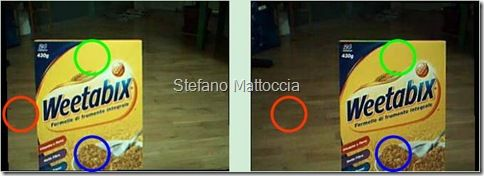（2） 平滑表面的镜面反射（3） 投影缩减（Foreshortening）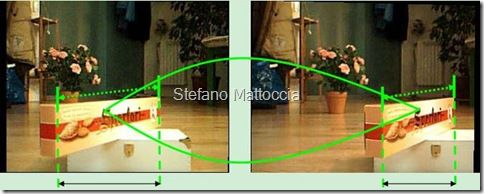（4） 透视失真（Perspective distortions）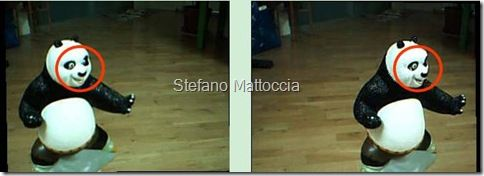（5） 低纹理（Low texture）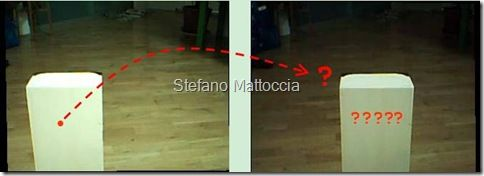（6） 重复纹理（Repetitive/ambiguous patterns）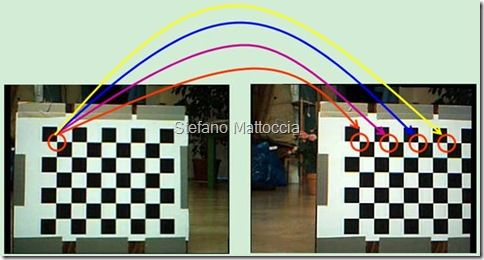（7） 透明物体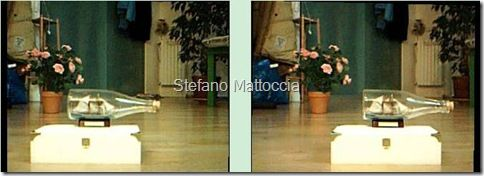（8） 重叠和非连续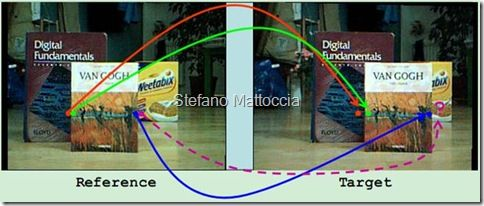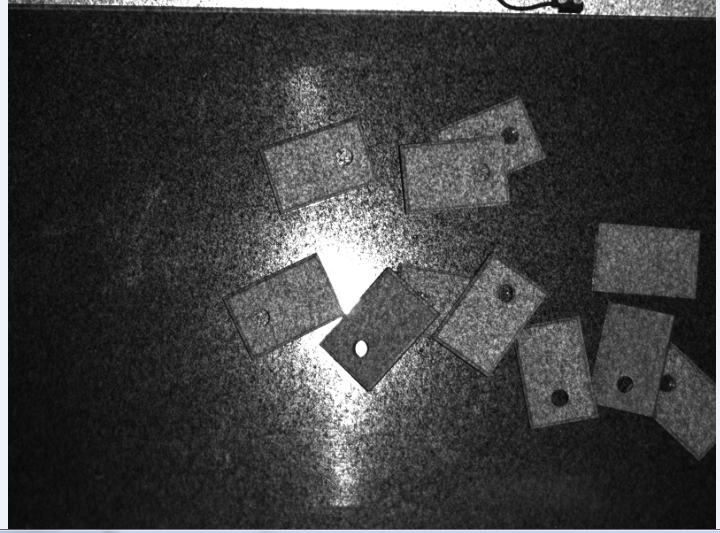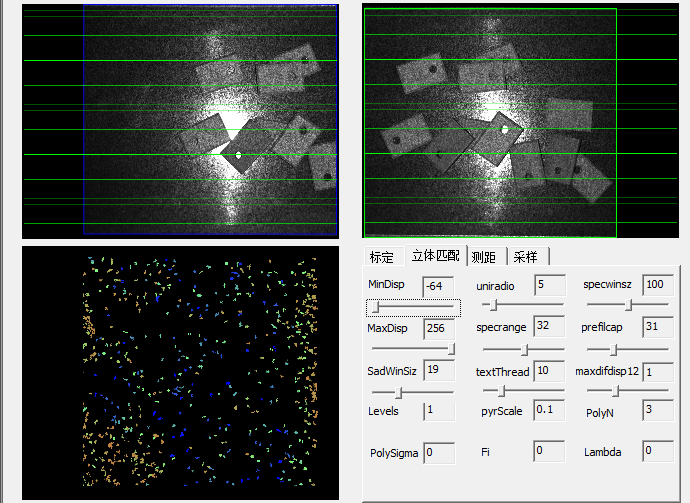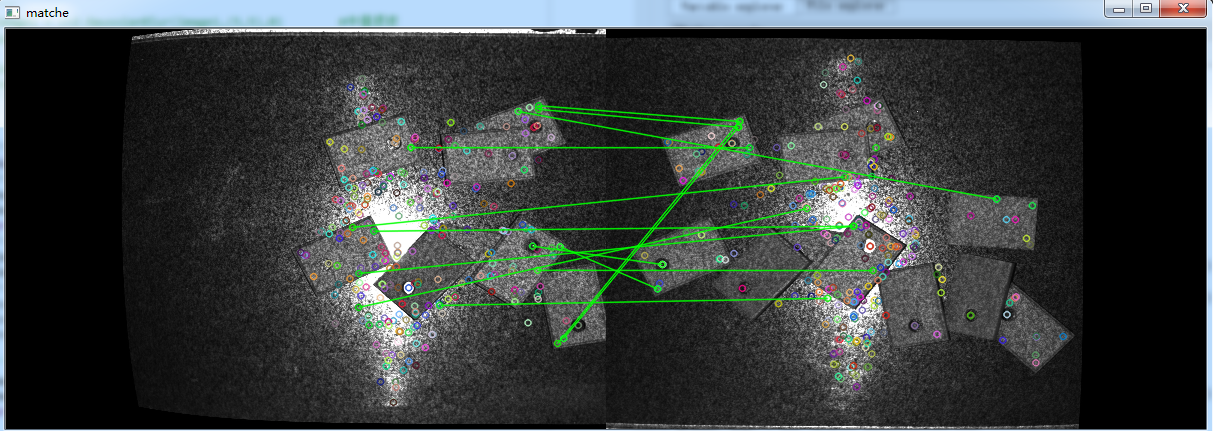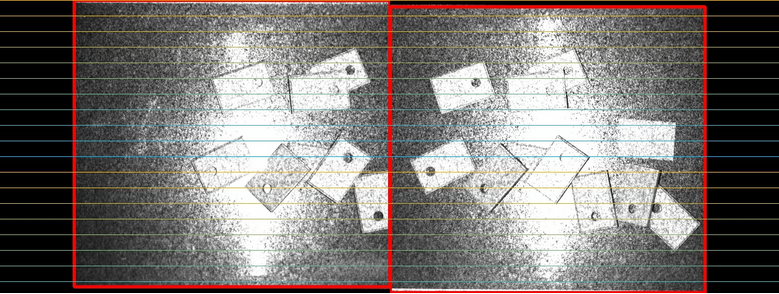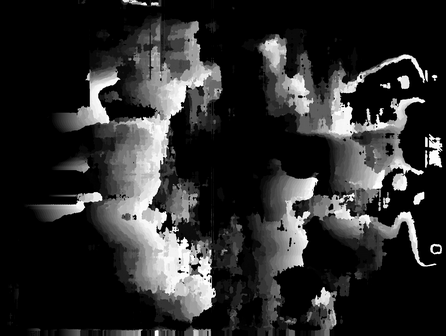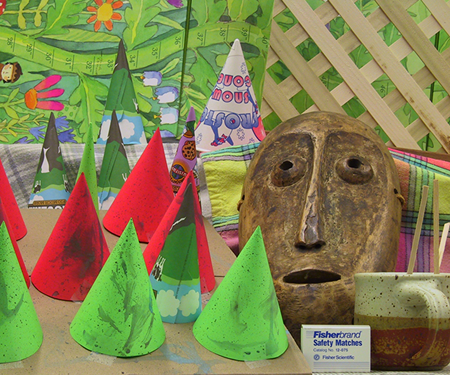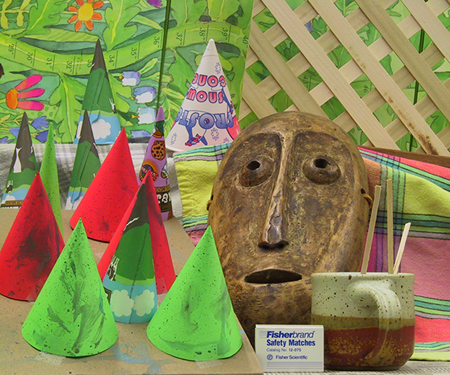最小二乘解（Least-squares Minimization ）

Stereo Vision:Algorithms and Applications(英文PPT，讲得很好)

【opencv】双目视觉下空间坐标计算/双目测距 6/13更新(推荐)

双目测距数学原理详解

opencv双目测距实现

【立体视觉】双目立体标定与立体校正

旋转矩阵(Rotate Matrix)的性质分析

本质矩阵和基础矩阵的区别是什么？

Bouguet极线校正进一步理解

双目定标与双目校正(推荐)

点积与叉乘的运算与物理意义

机器视觉学习笔记（8）——基于OpenCV的Bouguet立体校正

【OpenCV】双目测距（双目标定、双目校正和立体匹配）

双目标定，匹配的笔记

双目测距与三维重建的OpenCV实现问题集锦（一）图像获取与单目定标

双目测距与三维重建的OpenCV实现问题集锦（二）双目定标与双目校正

双目测距与三维重建的OpenCV实现问题集锦（三）立体匹配与视差计算

双目测距与三维重建的OpenCV实现问题集锦（四）三维重建与OpenGL显示

双目匹配值SGBM算法 (Semi-global matching) 半全局匹配算法

OpenCV3.4两种立体匹配算法效果对比

立体视觉-opencv中立体匹配相关代码

真实场景的双目立体匹配（Stereo Matching）获取深度图详解

学习OpenCV(4) 基于OpenCV的双目测距程序(推荐、附有代码)

【双目视觉探索路5】分析整理Learning OpenCV3书中立体标定、校正以及对应代码（3）之SGBM算法

计算机视觉 opencv双目视觉 立体视觉 三维重建

posted @ 2018-07-26 22:47  大奥特曼打小怪兽  阅读(26785)  评论(11编辑  收藏  举报